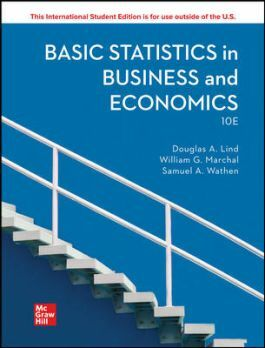•# Basic Statistics in Business and Economics, 10th Edition, 9781260597578

RM 140.00
- +

10th Edition

1260597571 · 9781260597578

Basic Statistics in Business & Economics provides students majoring in management, marketing, finance, accounting, economics, and other fields of business administration with an introductory survey of descriptive and inferential statistics. Many examples and exercises that focus on business applications are used to illustrate the application of statistics, but also relate to the current world of the college student. A previous course in statistics is not necessary, and the mathematical requirement is first-year algebra.
Students are given every step needed to be successful in a basic statistics course. This step-by-step approach enhances performance, accelerates preparedness, and significantly improves motivation. Understanding the concepts, seeing and doing plenty of examples and exercises, and comprehending the application of statistical methods in business and economics are the focus of this book.
Today, the practice of data analytics is widely applied to big data. The practice of data analytics requires skills and knowledge in several areas. Computer skills are needed to process large volumes of information. Analytical skills are needed to evaluate, summarize, organize, and analyze the information. Critical thinking skills are needed to interpret and communicate the results of processing the information. This text supports the development of basic data analytical skills with the end of each chapter sections called Data Analytics providing the instructor and student with opportunities to apply statistical knowledge and statistical software to explore several business environments. Interpretation of the analytical results is an integral part of these exercises.

Chapter 1: What is Statistics?

Chapter 2: Describing Data: Frequency Tables, FrequencyDistributions, and Graphic Presentation

Chapter 3: Describing Data: Numerical Measures

Chapter 4: Describing Data: Displaying and Exploring Data

Chapter 5: A Survey of Probability Concepts

Chapter 6: Discrete Probability Distributions

Chapter 7: Continuous Probability Distributions

Chapter 8: Sampling, Sampling Methods, and the Central LimitTheorem

Chapter 9: Estimation and Confidence Intervals

Chapter 10: One-Sample Tests of Hypothesis

Chapter 11: Two-Sample Tests of Hypothesis

Chapter 12: Analysis of Variance

Chapter 13: Correlation and Linear Regression

Chapter 14: Multiple Regression Analysis

Chapter 15: Nonparametric Methods: Nominal-Level HypothesisTests